﻿ Finite Element Galerkin’s Approach for Viscous Incompressible Fluid Flow through a Porous Medium in Coaxial Cylinders

### Finite Element Galerkin’s Approach for Viscous Incompressible Fluid Flow through a Porous Medium in ...

Anil Kumar, SP Agrawal, Pawan Preet KaurOPEN ACCESSPEER-REVIEWED

## Finite Element Galerkin’s Approach for Viscous Incompressible Fluid Flow through a Porous Medium in Coaxial Cylinders

Anil Kumar1,, SP Agrawal2, Pawan Preet Kaur3

1Department of Applied Mathematics, World Institute of Technology Sohna, Gurgaon, India

2Department of Civil Engineering, World Institute of Technology Sohna, Gurgaon, India

3Deparment of Applied Mathematics, Lyallpur Khalsa College Engineering Jalandhar Punjab

### Abstract

In this paper, we are considering viscous incompressible fluid flow through a porous medium between two coaxial cylinders. The governing equations have been solved by using Finite element Galekin’s approach. The velocity and temperature profiles of the flow are computed numerically and their behaviours are discussed by graphs for different values of the parameters.

### At a glance: Figures

123
Prev Next

• Kumar, Anil, SP Agrawal, and Pawan Preet Kaur. "Finite Element Galerkin’s Approach for Viscous Incompressible Fluid Flow through a Porous Medium in Coaxial Cylinders." Journal of Mathematical Sciences and Applications 1.3 (2013): 39-42.
• Kumar, A. , Agrawal, S. , & Kaur, P. P. (2013). Finite Element Galerkin’s Approach for Viscous Incompressible Fluid Flow through a Porous Medium in Coaxial Cylinders. Journal of Mathematical Sciences and Applications, 1(3), 39-42.
• Kumar, Anil, SP Agrawal, and Pawan Preet Kaur. "Finite Element Galerkin’s Approach for Viscous Incompressible Fluid Flow through a Porous Medium in Coaxial Cylinders." Journal of Mathematical Sciences and Applications 1, no. 3 (2013): 39-42.

 Import into BibTeX Import into EndNote Import into RefMan Import into RefWorks

### 1. Introduction

The important applications of MHD have been reported relating to the MHD generators, MHD pumps, nuclear reactors and MHD marine propulsion. The increasing cost of energy has lead technologists to examine measures which could considerably reduce the usage of the natural source energy. Heat transfers in magnetic thermal insulation within vertical cylinders annuli provide us insight into the mechanism of energy transport and enable engineers to use insulation more efficiently. Stephenson (1969) studied magnetohydrodynamic flow between rotating co-axial disks. Gupta et al. (1979) studied laminar free convective flow with and without heat sources through coaxial circular pipes. Varshney (1979) persuaded unsteady MHD flow of fluid through a porous medium in a circular pipe. Rath and Jena (1979) investigated fluctuating fluid between two coaxial cylinders. Gupta and Sharma (1981) persuaded the MHD flow of a conducting viscous incompressible fluid through porous media in equilateral triangular tube Pathak and Upadhyay (1981) investigated stability of dusty flow between two rotating coaxial cylinders. Shadday et al. (1983) studied the flow of an incompressible fluid in a partially filled rapidly rotating cylinder with a differentially rotating end cap. Pillai et al. (1989) discussed flow of a conducting fluid between two coaxial rotating porous cylinders bounded by a permeable bed. Javadpour and Bhattacharya (1991) discussed an axial flow in a rotating coaxial rheometer system bingham plastic. Gupta and Gupta (1996) investigated steady flow of an elastico-viscous fluid in porous coaxial circular cylinders. Abourabia et al. (2002) studied an unsteady heat transfer of a monatomic gas between two coaxial circular cylinders.

Ratnam and Malleswari (2004) investigated convection flow through a porous medium in a coaxial cylinder. Krishna and Rao (2005) studied finite element analysis of viscous flow through a porous medium in a triangular duct. Nobre et al. (2006) studied the effects of interfaces in the propagation of the energy by optical modes in coaxial cylinders. Mazumdar and Deka (2007) investigated MHD flow past an impulsively started infinite vertical plate in the presence of thermal radiation. Oysu (2007) discussed Finite element and boundary element contact stress analysis with remeshing technique. Srinivasacharya and Shifera (2008) investigated numerical solution to the MHD flow of micropolar fluid between two concentric porous cylinders. Hossain et al. (2009) investigated the f. Makinde et al. (2009) studied MHD viscous flow in a rotating porous medium cylindrical annulus with an applied radial field.

In this paper, we have analysed the free and forced convection of viscous fluid flow in coaxial cylinders taking into account the viscous dissipation. The governing equations have been solved by using Galerkin’s approach. The velocity and temperature profiles of the fluid flow are computed numerically and their behaviour is discussed by graphs for different values of the governing parameters.

### 2. Mathematical Analysis

In the present investigation, we are considering a the fully developed steady laminar free and forced convective flow of viscous incompressible fluid through a porous medium in between two vertical coaxial cylinders. Supposebe the cylindrical coordinate system such that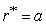andare the radii of inner and outer cylinders respectively. Assuming that the pipes are long enough so that all the physical quantities are independent ofand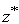. The motion being rotationally symmetric so the azimuthal velocity is zero. The governing equations in non-dimensional form are given by,(1)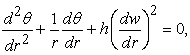(2)

wherew is the non-dimensional velocity component along z-axis,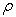the density of the fluid, P the fluid pressure, T the non-dimensional temperature of the fluid,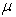the coefficient of the viscosity,the specific heat at constant pressure,the kinematic viscosity,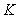the permeability of porous medium,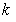the coefficient of thermal conductivity,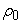the equilibrium density,the equilibrium temperature,the equilibrium pressure and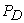the dynamic pressure.

K1 Porosity ,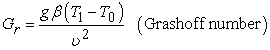and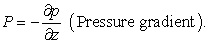The hydrostatic balance equation gives(3)

The corresponding boundary conditions are:(4)

### 3. Numerical Method

In order to solve equations (1) and (2) under the boundary conditions (4), the finite element method of Galerkin’s approach has been used. In Galerkin’s method the weight function is equal to the approximation function. Suppose domainof the radial direction is divided into n subintervals and length of each interval is, where(the gap duct between two coaxial cylinders) Kumar et al .

The Galerkin’s scheme is defined by(5)

whereis residual function and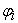weighting function.

From equation (1), we have residual functionWe use the Galerkin’s integral in the form(6)

whereis the volume of the annulus bounded by coaxial cylinders of unit length. The weak form of the equation (6) is given below:(7)

Integrating equation (7) by parts, we get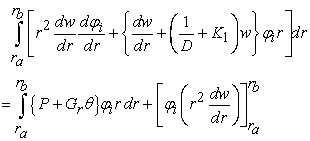or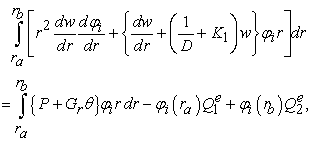here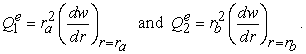Similarly, weak form of equation (2) can be expressed as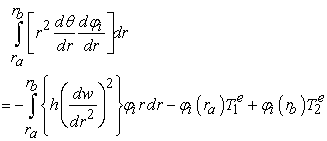where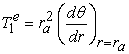and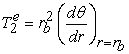.

Let us assume that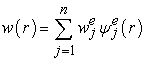and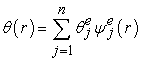are the approximate solutions of the equations (1) and (2). Assuming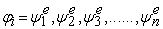(weight function is equal to the approximation function). Therefore the Finite element model for velocity and temperature profiles are given by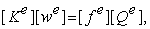(8)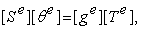(9)

where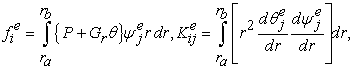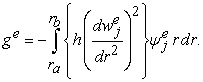Taking the approximate function as a linear interpolation function of the form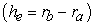,The equations (8) and (9) are the standard Galerkin’s finite elements equations. Their solutions can be obtained by Predictor-Corrector method.

### 4. Results and Discussion

The free and forced convection flow of viscous incompressible fluid through coaxial cylinders is discussed taking into account the viscous dissipation effects.

Here>0 indicates that the temperature of the outer boundary is higher than the inner in a coaxial cylinder duct or vice versa (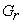<0).

Figure 1. Velocity Profiles for Various Number of Grashoff Number Gr (s=1.1, P=1, D-1=10000, h=0.001, K1=0.732)

Figures 1 and 5 show that the axial velocity of the fluid be maximum at the mid region while velocity profile upwards for>0 and downwards for<0. From these figures we observe that the enhancement in the velocity profile depends upon the nature of the gap duct between two coaxial cylinders. The magnitude of the axial velocity in narrow gap duct is less than that of wider gap duct for a given Grashoff number(). Figures 2 and 6 depict that the velocity profile increases with the increasing values of Darcy’s parameterin both narrow and wider gap duct while the enhancement in the wider gap duct is higher than to the narrow gap duct. Figure 3 depicts that the temperature profiles for various values of () in a narrow gap duct. Figure 4 depicts that the temperature profiles for various values of Darcy’s parameter. The temperature profile increases with the decrease in the permeability of the porous medium and effect of magnetic field. Figure 7 and Figure 8 depict that the temperature profiles for various values of Grashoff number () and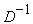respectively in wide gap duct of the coaxial cylinders. The temperature assumes positive values faster as we move from the inner boundary to outer boundary than in the case of narrow gap duct. The nature of temperature profile in wider gap duct of coaxial cylinders for positive or negative values of,is similar to that of the corresponding case in a narrow gap duct of the coaxial cylinders. The present algorithm is economic and efficient having a sharp convergence.

Figure 8. Temperature Profiles for Different Values D-1, (s=1.5, P=1, Gr=400, h=0.001, K1=1)

### References

  M. Gupta, G.K. Dubey and H.S. Sharma, “Laminar free convective flow with and without heat sources through coaxial circular pipes,” Indian Journal of Pure and Applied Mathematics, vol. 10(7), pp 792-799, 1979.In article  R.S. Rath and G. Jena, “Fluctuating fluid between two coaxial cylinders,” Indian Journal of Pure and Applied Mathematics, vol. 10(11), pp 1405-1414, 1979.In article  R.S. Pathak and B.N. Upadhyay, “Stability of dusty flow between two rotating coaxial cylinders,” Def. Science Journal, vol. 31(2), pp 155-165, 1981.In article  K. J. Pillai, S.V.K Verma and M.S. Babu, “Flow of a conducting fluid between two coaxial rotating porous cylinders bounded by a permeable bed,” Indian Journal of Pure and Applied Mathematics, vol. 20(5), pp 526-536, 1989.In article  S.H. Javadpour and S.N. Bhattacharya, “Axial flow in a rotating coaxial rheometer system bingham plastic,” J. Sci. I.R. Iran, vol. 2(3-4), pp 151-158, 1991.In article  R.K. Gupta and K. Gupta, “Steady flow of an elastico-viscous fluid in porous coaxial circular cylinders,” Indian Journal of Pure and Applied Mathematics, vol. 27(4), pp 423-434, 1996.In article  B. Barman, “Flow of a newtonian fluid past an impervious sphere embedded in a porous medium,” Indian Journal of Applied and Pure Mathematics, vol. 27(12), pp 1249-1256, 1996.In article  A.M. Abourabia, M.A. Mahmoud and W.S.A. Kareem, “Unsteady heat transfer of a monatomic gas between two coaxial circular cylinders,” Journal of Applied Mathematics, vol. 2(3), pp 141-161, 2002.In article CrossRef  L. Ratnam and D. Malleswari, Convection flow through a porous medium in a coaxial cylinder, Bulletin of Pure and Applied Sciences, vol. 24E (1), pp 145-152, 2004.In article  D.V. Krishna and P.N. Rao, “Finite element analysis of viscous flow through a porous medium in a triangular duct,” Bulletin of Pure and Applied Sciences, vol. 24E (1), pp 1-16, 2005.In article  E.F. Nobre, R.S.T. Moretzsonh, R.C. Vilela, G.A. Farias and N.S. Almeida, “Study of effects of interfaces in the propagation of the energy by optical modes in coaxial cylinders,” Brazilian Journal of Physics, vol. 36(3B), pp 967-970, 2006.In article CrossRef  M.K. Mazumdar and R.K. Deka, “MHD flow past an impulsively started infinite vertical plate in the presence of thermal radiation,” Romanian Journal of Physics, vol. 52(5-7), pp 565-573, 2007.In article  C. Oysu, “Finite element and boundary element contact stress analysis with remeshing technique,” Applied Mathematical Modelling, vol 31, pp 2744-2753, 2007.In article CrossRef  D. Srinivasacharya and M. Shifera, “Numerical solution to the MHD flow of micropolar fluid between two concentric porous cylinders,” Indian Journal of Applied Mathematics and Mechanics, vol. 4(2), pp 77-86, 2008.In article  O.D. Makinde, O.A. Beg and H.S. Takhar, “Magnetohydrodynamic viscous flow in a rotating porous medium cylindrical annulus with an applied radial field,” Indian Journal of Applied Mathematics and Mechanics, vol. 5(6), pp 68-81, 2009.In article  F. Hacivelioglu and A. Buyukaksoy, “Analysis of coaxial waveguide with finite-length impedance leading in the inner and outer conductors, Mathematical Problems in Engineering, vol. 55, pp 1-18, 2009.In article CrossRef  Anil Kumar , C L Varshney and Sajjan Lal Perturbation Technique of MHD Free Convection Flow through Infinite Vertical Porous Plate with Constant Heat Flux, International Journal of Mathematical Modeling and Physical Sciences Vol. 01, Issue 02, June 2013, pp 1-5.In article